x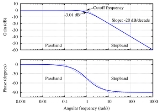Encyclopedia
One decade is a factor of 10 difference between two numbers (an order of magnitude
Order of magnitude
An order of magnitude is the class of scale or magnitude of any amount, where each class contains values of a fixed ratio to the class preceding it. In its most common usage, the amount being scaled is 10 and the scale is the exponent being applied to this amount...

difference) measured on a logarithmic scale
Logarithmic scale
A logarithmic scale is a scale of measurement using the logarithm of a physical quantity instead of the quantity itself.A simple example is a chart whose vertical axis increments are labeled 1, 10, 100, 1000, instead of 1, 2, 3, 4...

. It is especially useful when referring to frequencies and when describing frequency response
Frequency response
Frequency response is the quantitative measure of the output spectrum of a system or device in response to a stimulus, and is used to characterize the dynamics of the system. It is a measure of magnitude and phase of the output as a function of frequency, in comparison to the input...

of electronic systems
Electronics
Electronics is the branch of science, engineering and technology that deals with electrical circuits involving active electrical components such as vacuum tubes, transistors, diodes and integrated circuits, and associated passive interconnection technologies...

, such as audio amplifier
Audio amplifier
An audio amplifier is an electronic amplifier that amplifies low-power audio signals to a level suitable for driving loudspeakers and is the final stage in a typical audio playback chain.The preceding stages in such a chain are low power audio amplifiers which perform tasks like pre-amplification,...

s and filters
Electronic filter
Electronic filters are electronic circuits which perform signal processing functions, specifically to remove unwanted frequency components from the signal, to enhance wanted ones, or both...

.

## Calculations

The factor-of-ten in a decade can be in either direction: so one decade up from 100 Hz is 1000 Hz, and one decade down is 10 Hz. The factor-of-ten is what is important, not the unit used, so 3.14 rad/s is one decade down from 31.4 rad/s.

To determine the number of decades between two frequencies, use the logarithm
Logarithm
The logarithm of a number is the exponent by which another fixed value, the base, has to be raised to produce that number. For example, the logarithm of 1000 to base 10 is 3, because 1000 is 10 to the power 3: More generally, if x = by, then y is the logarithm of x to base b, and is written...

of the ratio of the two values: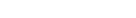decades
How many decades is it from 3.2 GHz to 4.7 MHz?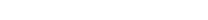decades
How many decades is one octave
Octave (electronics)
In electronics, an octave is a doubling or halving of a frequency. The term is derived from the musical octave which similarly describes such frequency ratios, but the prefix octa-, denoting eight, has no significance in physics...

?
One octave is a factor of 2, so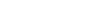decades per octave

To find out what frequency is a certain number of decades from the original frequency, multiply by appropriate powers of 10:
What is 3 decades down from 220 Hz?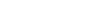Hz
What is 1.5 decades up from 10?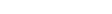To find out the size of a step for a certain number of frequencies per decade, raise 10 to the power of the inverse of the number of steps:
What is the step size for 30 steps per decade?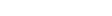- or each step is 7.9775% larger than the last.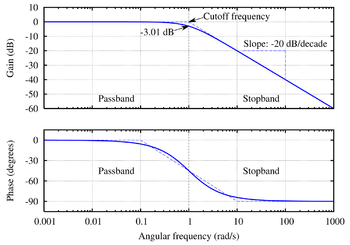## Graphical representation and analysis

Logarithmic scale
A logarithmic scale is a scale of measurement using the logarithm of a physical quantity instead of the quantity itself.A simple example is a chart whose vertical axis increments are labeled 1, 10, 100, 1000, instead of 1, 2, 3, 4...

, rather than unit steps (steps of 1) or other linear
Linear
In mathematics, a linear map or function f is a function which satisfies the following two properties:* Additivity : f = f + f...

scale, are commonly used on the horizontal axis when representing the frequency response of electronic circuits in graphical form, such as in Bode plot
Bode plot
A Bode plot is a graph of the transfer function of a linear, time-invariant system versus frequency, plotted with a log-frequency axis, to show the system's frequency response...

s, since depicting large frequency ranges on a linear scale is often not practical. For example, an audio amplifier
Audio amplifier
An audio amplifier is an electronic amplifier that amplifies low-power audio signals to a level suitable for driving loudspeakers and is the final stage in a typical audio playback chain.The preceding stages in such a chain are low power audio amplifiers which perform tasks like pre-amplification,...

will usually have a frequency band ranging from 20 Hz to 20 kHz and representing the entire band using a decade log scale is very convenient. Typically the graph for such a representation would begin at 1 Hz (100) and go up to perhaps 100 kHz (105), to comfortably include the full audio band in a standard-sized graph paper, as shown below. Whereas in the same distance on a linear scale, with 10 as the major step-size, you might only get from 0 to 50.
Electronic frequency responses are often described in terms of "per decade". The example Bode plot shows a slope of -20 dB
Decibel
The decibel is a logarithmic unit that indicates the ratio of a physical quantity relative to a specified or implied reference level. A ratio in decibels is ten times the logarithm to base 10 of the ratio of two power quantities...

/decade in the stopband, which means that for every factor-of-ten increase in frequency (going from 10 rad/s to 100 rad/s in the figure), the gain decreases by 20 dB.

• Octave
Octave (electronics)
In electronics, an octave is a doubling or halving of a frequency. The term is derived from the musical octave which similarly describes such frequency ratios, but the prefix octa-, denoting eight, has no significance in physics...

• Frequency
Frequency
Frequency is the number of occurrences of a repeating event per unit time. It is also referred to as temporal frequency.The period is the duration of one cycle in a repeating event, so the period is the reciprocal of the frequency...

• Logarithm
Logarithm
The logarithm of a number is the exponent by which another fixed value, the base, has to be raised to produce that number. For example, the logarithm of 1000 to base 10 is 3, because 1000 is 10 to the power 3: More generally, if x = by, then y is the logarithm of x to base b, and is written...

• Bode plot
Bode plot
A Bode plot is a graph of the transfer function of a linear, time-invariant system versus frequency, plotted with a log-frequency axis, to show the system's frequency response...

• Electronic filter
Electronic filter
Electronic filters are electronic circuits which perform signal processing functions, specifically to remove unwanted frequency components from the signal, to enhance wanted ones, or both...

• Frequency response
Frequency response
Frequency response is the quantitative measure of the output spectrum of a system or device in response to a stimulus, and is used to characterize the dynamics of the system. It is a measure of magnitude and phase of the output as a function of frequency, in comparison to the input...

• Decibel
Decibel
The decibel is a logarithmic unit that indicates the ratio of a physical quantity relative to a specified or implied reference level. A ratio in decibels is ten times the logarithm to base 10 of the ratio of two power quantities...

• Graph paper
Graph paper
Graph paper, graphing paper, grid paper or millimeter paper is writing paper that is printed with fine lines making up a regular grid. The lines are often used as guides for plotting mathematical functions or experimental data and drawing diagrams. It is commonly found in mathematics and...